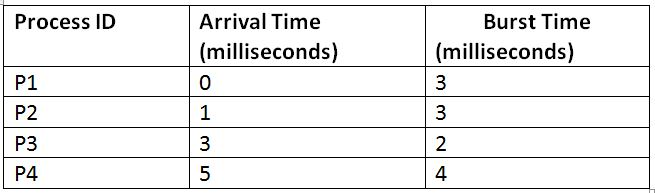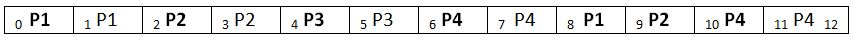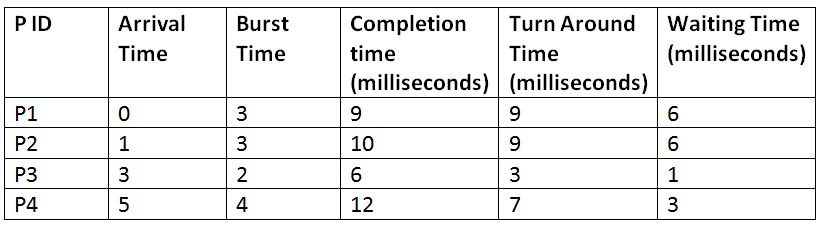# Round Robin Scheduling in Operating System

In this article, we are going to study about Round Robin Scheduling, which is one of the well-known and widely used process scheduling algorithms. We are going to learn about the working of this algorithm, the mode in which it functions and will also take a look at one of its examples for understanding the working of this algorithm.
Submitted by Monika Sharma, on June 27, 2019

Round Robin scheduling algorithm is a type of preemptive type of scheduling used by the operating system for scheduling the processes. In the Round Robin scheduling algorithm, a time quantum is decided which remains constant throughout the execution of all processes. Each process executes only for this much time. If within this time, the process completes its execution, then it is terminated. Else, it waits for its turn for again getting the processor for the same time quantum, and this process continues. Let us understand it further with the help of an example.

Suppose we have 4 processes: P1, P2, P3, and P4, with the following properties:And suppose that the time quantum decided is 2 units. So, the execution of these processes while following the Round Robin Scheduling will be as follows:

Gant Chart:Explanation:

• P1 runs for 2 units from 0 to 2, and now it needs the 1-time unit more for completion.
• P2 runs from 2 to 4 and it also now it also needs only a one-time unit more for completion.
• P3 runs from 4-6 and within this time quantum itself, it has been completed.
• Now, P4 runs from 6 to 8 and now it needs 2-time units more.
• Now, P1 is again provided the processor for 2-time units as per the decided time quantum, but its execution completes in 1-time unit itself. So, without any delay, the subsequent process is executed.
• Same as P1, P2 also gets completed in 1-time unit itself.
• Then P4 is provided the processor and it completes its execution.```    Total Turn-Around Time = 9 + 9 + 3 + 7
= 28 milliseconds
Average Turn-Around Time= Total Turn-Around Time / Total No. of Processes
= 28 / 4
= 7 milliseconds

Total Waiting Time = 6 + 6 + 1 + 3
= 16 milliseconds
Average Waiting Time = Total Waiting Time / Total No. of Processes
= 16 / 4
= 4 milliseconds
```

What's New

Top Interview Coding Problems/Challenges!

Languages: » C » C++ » C++ STL » Java » Data Structure » C#.Net » Android » Kotlin » SQL
Web Technologies: » PHP » Python » JavaScript » CSS » Ajax » Node.js » Web programming/HTML
Solved programs: » C » C++ » DS » Java » C#
Aptitude que. & ans.: » C » C++ » Java » DBMS
Interview que. & ans.: » C » Embedded C » Java » SEO » HR
CS Subjects: » CS Basics » O.S. » Networks » DBMS » Embedded Systems » Cloud Computing
» Machine learning » CS Organizations » Linux » DOS
More: » Articles » Puzzles » News/Updates